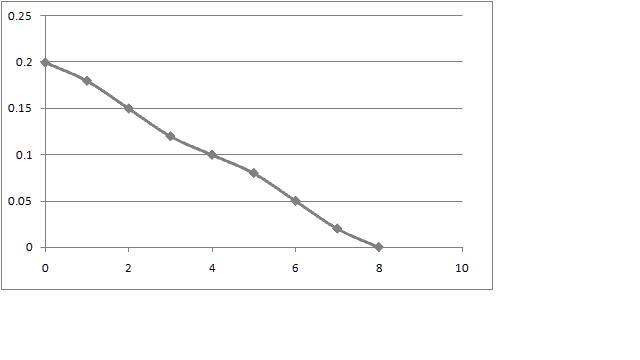# Determine from the graph the speed of the car

## Homework Statement

A toy car of mass 0.5 kg works with a wound spring that exerts a force as shown in the table below.The car was initially at rest. The spring is wound at the car set in motion on a horizontal surface.

t = 0 s ------> F = 0.2 N
t = 1 s ------> F = 0.18 N
t = 2 s ------> F = 0.15 N
t = 3 s ------> F = 0.12 N
t = 4 s ------> F = 0.1 N
t = 5 s ------> F = 0.08 N
t = 6 s ------> F = 0.05 N
t = 7 s ------> F = 0.02 N
t = 8 s ------> F = 0 N

a. Plot the graph of force vs time using the table above. Determine from the graph the speed of the car 8 s after being released.

b. The car then crashes into a stationary car of mass 1.5 kg and is stuck to the second car. Calculate the speed of the two cars after collision. Show if the collision is elastic or not

## Homework Equations

1. p = Ft
2. conservation of momentum

3. attempt at a solution
a.I'm not sure how to find the speed from the graph. The best I can try is I assume the graph is triangle, then I find the area which is equal to change in momentum.

Area = change in momentum
0.5*0.2*8 = mv
v = 1.6 ms-1

b. m1u1+m2u2=(m1+m2)V
0.5*1.6 = (0.5 + 1.5) V
V = 0.4 ms-1

The collision is perfectly inelastic

Am I right ?

Thanks

## Answers and Replies

rock.freak667
Homework Helper

your theory looks correct to me.

Hi rock.freak667

Thanks a lot !!